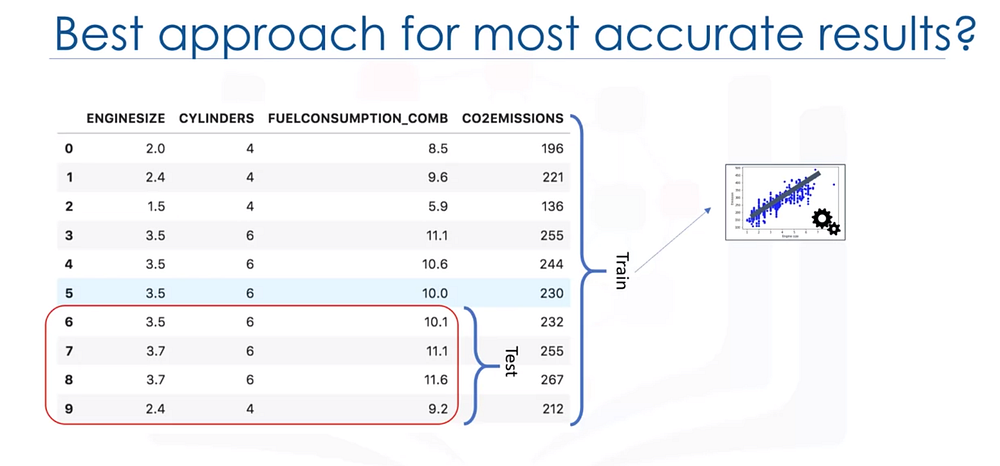# In this blog, I’ll be covering model evaluation.

Original article was published on Artificial Intelligence on MediumIn this blog, I’ll be covering model evaluation. So let’s get started. The goal of regression is to build a model to accurately predict an unknown case. To this end, we have to perform regression evaluation after building the model. I’ll introduce and discuss two types of evaluation approaches that can be used to achieve this goal. These approaches are

• Train and test on the same dataset
• Train/test split.

We’ll talk about what each of these are, as well as the pros and cons of using each of these models. Also, we’ll introduce some metrics for accuracy of regression models.

Let’s look at the first approach. When considering evaluation models, we clearly want to choose the one that will give us the most accurate results. So, the question is, how can we calculate the accuracy of our model? In other words, how much can we trust this model for prediction of an unknown sample using a given dataset and having built a model such as linear regression? One of the solutions is to select a portion of our dataset for testing. For instance, assume that we have 10 records in our dataset.